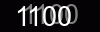# Export and import images to PDF file in C# and VB.NET

PDF .Net supports exporting images from the PDF file in JPEG, BMP, PNG or TIFF image formats.

The following example shows how you can export single image from the PDF file.

#### Complete code

``````using System;
using SautinSoft.Pdf;
using System.IO;

class Program
{
static void Main()
{

// This property is necessary only for licensed version.
//SautinSoft.Pdf.Serial = "XXXXXXXXXXX";

// Iterate through PDF pages and through each page's content elements.
foreach (var page in document.Pages)
foreach (var contentElement in page.Content.Elements.All())
if (contentElement.ElementType == PdfContentElementType.Image)
{
// Export an image content element to selected image format.
var imageContent = (PdfImageContent)contentElement;
imageContent.Save("ExportImages.jpeg");
return;
}
}
}
``````
```
```

``````

### Import images

PDF .Net supports importing images in a PDF file from your C# or VB.NET application.

The following example shows how you can easily create a PDF document and import an image to its first page.

#### Complete code

``````using System;
using SautinSoft.Pdf;
using System.IO;

class Program
{
static void Main()
{

// This property is necessary only for licensed version.
//SautinSoft.Pdf.Serial = "XXXXXXXXXXX";

using (var document = new PdfDocument())
{

// Load the image from a file.

// Set the location of the bottom-left corner of the image.
// We want the top-left corner of the image to be at location (50, 50)
// from the top-left corner of the page.
// NOTE: In PDF, location (0, 0) is at the bottom-left corner of the page
// and the positive y axis extends vertically upward.
double x = 50, y = page.CropBox.Top - 50 - image.Size.Height;

// Draw the image to the page.
page.Content.DrawImage(image, new PdfPoint(x, y));

document.Save("Stockholm.pdf");
}
}
}
``````
```
```

``````

### Image positioning and transformations

The following example shows how to position and apply various transformations, such as rotation or scaling, to an image drawn on a PDF page.

#### Complete code

``````using System;
using SautinSoft.Pdf;
using System.IO;

class Program
{
static void Main()
{

// This property is necessary only for licensed version.
//SautinSoft.Pdf.Serial = "XXXXXXXXXXX";

using (var document = new PdfDocument())
{

// Load the image from a file.

double margin = 50;

// Set the location of the first image in the top-left corner of the page (with a specified margin).
double x = margin;
double y = page.CropBox.Top - margin - image.Size.Height;

// Draw the first image.
page.Content.DrawImage(image, new PdfPoint(x, y));

// Set the location of the second image in the top-right corner of the page (with the same margin).
x = page.CropBox.Right - margin - image.Size.Width;
y = page.CropBox.Top - margin - image.Size.Height;

// Initialize the transformation.
var transform = PdfMatrix.Identity;
// Use the translate operation to position the image.
transform.Translate(x, y);
// Use the scale operation to resize the image.
// NOTE: The unit square of user space, bounded by user coordinates (0, 0) and (1, 1),
// corresponds to the boundary of the image in the image space.
transform.Scale(image.Size.Width, image.Size.Height);
// Use the scale operation to flip the image horizontally.
transform.Scale(-1, 1, 0.5, 0);

// Draw the second image.
page.Content.DrawImage(image, transform);

// Set the location of the third image in the bottom-left corner of the page (with the same margin).
x = margin;
y = margin;

// Initialize the transformation.
transform = PdfMatrix.Identity;
// Use the translate operation to position the image.
transform.Translate(x, y);
// Use the scale operation to resize the image.
transform.Scale(image.Size.Width, image.Size.Height);
// Use the scale operation to flip the image vertically.
transform.Scale(1, -1, 0, 0.5);

// Draw the third image.
page.Content.DrawImage(image, transform);

// Set the location of the fourth image in the bottom-right corner of the page (with the same margin).
x = page.CropBox.Right - margin - image.Size.Width;
y = margin;

// Initialize the transformation.
transform = PdfMatrix.Identity;
// Use the translate operation to position the image.
transform.Translate(x, y);
// Use the scale operation to resize the image.
transform.Scale(image.Size.Width, image.Size.Height);
// Use the scale operation to flip the image horizontally and vertically.
transform.Scale(-1, -1, 0.5, 0.5);

// Draw the fourth image.
page.Content.DrawImage(image, transform);

document.Save("PositioningTransformations.pdf");
}
}
}
``````
```
```

``````

If you need a new code example or have a question: email us at support@sautinsoft.com or ask at Online Chat (right-bottom corner of this page) or use the Form below:#### Questions and suggestions from you are always welcome!

We are developing .Net components since 2002. We know PDF, DOCX, RTF, HTML, XLSX and Images formats. If you need any assistance with creating, modifying or converting documents in various formats, we can help you. We will write any code example for you absolutely free.